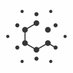A community of mathematicians say the equal sign is too rigid to capture many mathematical complexities. They're replacing equality with a new mathematics of equivalence.The equal sign is the bedrock of mathematics. But there is a growing community of mathematicians who regard the equal sign as math’s original error."There is a growing community of mathematicians who regard the equal sign as math’s original error." reports in on the movement toward category theory in mathematics.A community of mathematicians say the equal sign is too rigid to capture many mathematical complexities. They're replacing equality with a new mathematics of equivalence.With Category Theory, Mathematics Escapes From Equality Two monumental works have led many mathematicians to avoid the equal sign. Their goal: Rebuild the foundations of the discipline upon the looser relationship of “equivalence.”Genius has an important role in developing mathematics, but actually the knowledge itself is the result of the activity of a community [...] it’s the real goal of knowledge to become the knowledge of the community, not the knowledge of one or two persons.